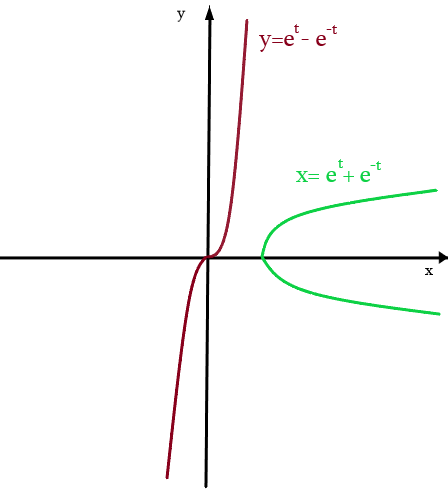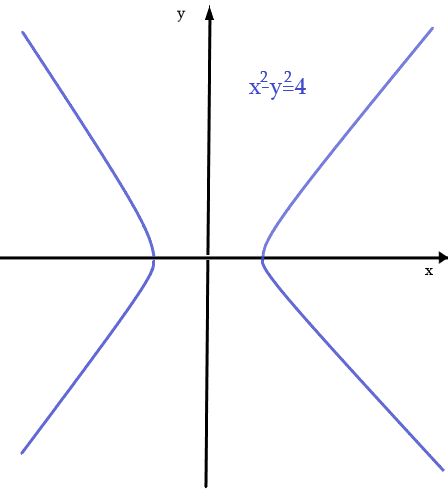# Compare the curves represented by the parametric equations. How do they differ? Sketch graphs. x...

## Question:

Compare the curves represented by the parametric equations. How do they differ? Sketch graphs.

{eq}\displaystyle x = e^t + e^{-t},\ y = e^t - e^{-t} {/eq}.

## Parametric Equations:

If {eq}x {/eq} and {eq}y {/eq} are continuous functions of {eq}t_1,t_2,\dots,t_n {/eq} on an open set {eq}D \subset \mathbb{R}^n {/eq}, then the equations

{eq}\begin{align} x=x(t_1,t_2,\dots,t_n)\\ y=y(t_1,t_2,\dots,t_n) \end{align} {/eq}

are called parametric equations and {eq}t_1,t_2,\dots,t_n {/eq} is called the parameters.

Note that both curves are linear combinations of the same functions that differ only in the sign of the function segment {eq}e^{-t} {/eq}.

• Since the function {eq}e^{\pm t} {/eq} is greater than zero, we also notice that {eq}x {/eq} is always a positive function, while in the curve {eq}y {/eq}:

{eq}\begin{align} &y> 0 \iff e^{t} > \frac{1}{e^t} \\ &e^{2t} > 1 \iff 2t>0 \\ & \implies t>0 \end{align} {/eq}

And vice versa, for t less than zero, the function takes negative values.

• On the other hand, look at the parity of these curves to determine their difference in the graph:

{eq}\begin{align} &x(-t) = e^{-t} + e^{t} = x(t) \\ &y(-t) = e^{-t} - e^{t} = -y(t) \end{align} {/eq}

Therefore, we see that {eq}x {/eq} is an even function and {eq}y {/eq} is an odd function

• Finally, if we subtract the squares of these two curves, we get:

{eq}\begin{align} x^2 - y^2 = e^{2t} + 2 + e^{-2t} - e^{2t} + 2 - e^{-2t} =4 \end{align} {/eq}

Which is a hyperbola.